Courses

# Chemical Equilibrium Introduction and Irreversible and Reversible Reactions (Part - 1) Chemistry Notes | EduRev

## Chemistry : Chemical Equilibrium Introduction and Irreversible and Reversible Reactions (Part - 1) Chemistry Notes | EduRev

The document Chemical Equilibrium Introduction and Irreversible and Reversible Reactions (Part - 1) Chemistry Notes | EduRev is a part of the Chemistry Course Physical Chemistry.
All you need of Chemistry at this link: Chemistry

In a chemical reaction, chemical equilibrium is the state in which both reactants and products are present in concentrations which have no further tendency to change with time, so that there is no observable change in the properties o f the system. Usually, this state results when the forward reaction proceeds at the same rate as the reverse reaction. The reaction rates of the forward and backward reactions are generally not zero, but equal. Thus, there are no net changes in the concentrations of the reactant(s) and product(s). Such a state is known as dynamic equilibrium.

1. Irreversible and Reversible Reactions

Irreversible reactions

Those reactions which proceeds in forward direction and reaches almost to completion are called Irreversible reactions.

For example: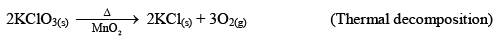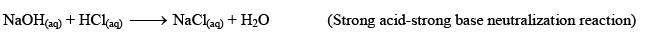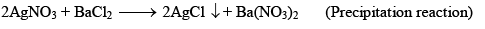Irreversible Reactions:

•  Always goes for completion.
•  Reactants are completely consumed in these react ions

Ex.: Ppt. reaction, NaCl + AgNO3→AgCl(s) + NaNO3

case, if we remove AgCl (which is the product), reaction will mo ve in forward direction].

Reversible reactions

Those reactions which proceed in both forward and backward directions and never reaches completion are called Reversible reactions.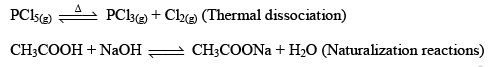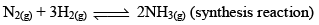But when Fe(s) is heated and water vapour is passed over it in open vessel, it is converted to Fe3O4(s) along with the evolution of hydrogen gas.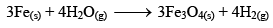And when Fe3O4 is reduced with hydrogen gas, it gives Fe(s) and H2OBut, if the react ion is carried out in close vessel, this react ion beco mes reversible.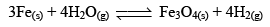Reversible reactions:

•  Never goes for completion
•  Irrespective of the fact whether the reaction has started by reactants or products.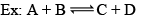If we remove C (which is product), reaction will again start and moves in forward direct ion to attain equilibrium again.

• These react ions can be initiated in any direction.
• Always takes place in a closed vessel.

2. State of Equilibrium

It has generally been observed that many changes (physical and chemical) do not proceed to completion when they are carried out in a closed container. Consider, for example, vaporisat ion of water,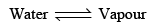At any temperature, vapourisation of water takes place, initially the concentration of water is much greater than the concentration of vapour, but with the progress of time, concentration of vapour increases whereas that of water remains constant and after a certain interval of time. There is no change in concentration of vapour, this state is known as state of physical equilibrium. In a similar way, this has also been found for chemical react ions, for example. When PCl5(g) is heated in a closed continue, its dissociation starts with the format ion of PCl3(g) and Cl2(g) are formed due to dissociation of PCl5(g). After a certain interval of time, the concentration o f PCl5(g), PCl3(g) and Cl2(g) each becomes constant. It does not mean that at this point of time, dissociation of PCl5(g) and its format ion fro m PCl3(g) and Cl2(g) has been stopped. Actually t he rate of dissociation o f PCl5 and the rate of formation of PCl5(g) becomes equal. This state is called the state of chemical equilibrium. So, the state of chemical equilibrium is dynamic.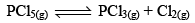This can be shown graphically.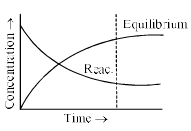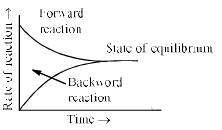So, “state of chemical equilibrium can be defined as the state when the rate of forward reaction becomes equal to rate of reverse reaction and the concentration of all the species becomes constant”.

3. Quantitative Aspects of Equilibrium: Law of Mass Action

Guldberg and Waage in 1807 gave this law and according to this law, “At constant temperature, the rate at which a substance reacts is directly proportional to its active mass and the rate at which a chemical reaction proceeds is directly proportional to the product of active masses of the reacting species”.

The term active mass of a reacting species is the effective, concentration or its activity (a) which is related to the molar concentration (C) as

a = f C

where f = activity coefficient. F < 1 but f increases with dilution and as V → ∞ i.e. C → 0, f → 1 i.e., a → C Thus at very low concentration the active mass is essentially the same as the molar concentration. It is generally expressed by enclosing the formula of the reacting species in a square bracket.

To illustrate the law of mass action, consider the following general reaction at constant temperature,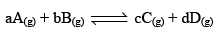Applying law of mass action,

Rate of forward reaction,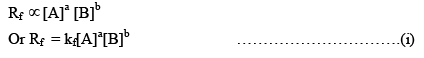Where k= rate constant of forward reaction

Similarly,

Rate of reverse reaction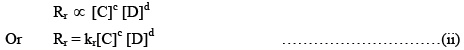Where k= rate constant of reverse reaction.

At equilibrium,

Rate of forward reaction = Rate of reverse reaction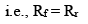So, from equation (i) and (ii), we get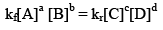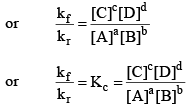where, Kc is the equilibrium constant in terms of molar concentration.

Illustration: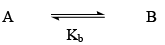The rate of disappearance of A is given as: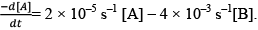Calculate the value of equilibrium constant.

Solution:

We know, at equilibrium concentration of each species present in the given equilibrium. Become constant.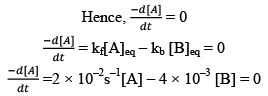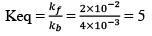Equilibrium Constant (Kp) in terms of Partial Pressure: Consider the same general reaction taking place at constant temperature,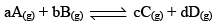From law of mass action,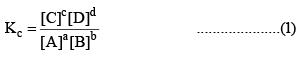From ideal gas equation

PV = nRT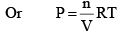At constant temperature,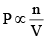Thus, we can say in a mixture of gases, Partial pressure of any component (say A)

P ∝[A]

Similarly, P ∝ [B]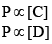So, equation (1) can be rewritten as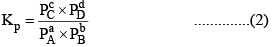All Equilibrium constants (Keq)-

• Kc
• Kp
• Kx
• Kn

Kx and Kn are not considered as equilibrium constants, because these can be varied without change in temp. for gases.

4. Relationship Between Kp and Kc

From the above, for the same general reaction at constant temperature.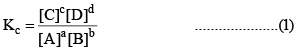And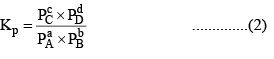From the ideal gas equation,

PV = nRT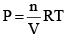So, PB = [B] RT; PD = [D]RT

Similarly, PA = [A]RT; PC = [C]RT

Substituting the values o f PA, PB, PC, and PD in equation (2), we get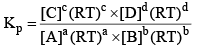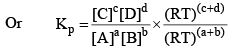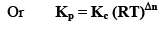Where, Δn = (c + d) - (a + b)

i.e;, Δn = sum of no. of moles of gaseous products-sum of no. of moles of gaseous reactants.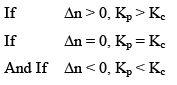Illustration: The value of Kp for the Reaction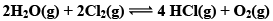is 0.03 atm at 4270C, when the partial pressure is expressed in atm. What is the value of Kc.

Solution: Firstly, consider species that are present in gaseous phase only. So, we can write Kp.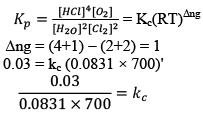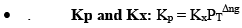5. Application of Law of Mass Action: Equilibrium Constant for Heterogeneous Equilibria Reversible Reactions:

• Homogeneous
• Heterogeneous

Homogeneous: Products and reactants are in same phase. Homogeneous gaseous equilibria are best classified into three categories i.e., in which no. of moles of product and reactant varies.

Case I: Homogeneous; Δng = 0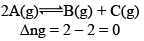In this reaction, each and every species present is in same phase. That’s why this reaction is under the category of homogeneous equilibria.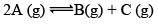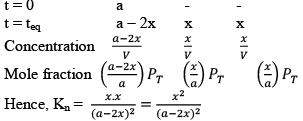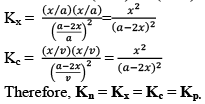PV = nRT (Ideal gas equation)

Here, V is constant. R is also constant.

P α n

Since no. of moles are constant i.e.  Initial = a

Final = a

Hence Palso remains constant and we will get the curve mention below.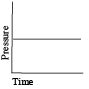Case II: Homogeneous:Δng > 0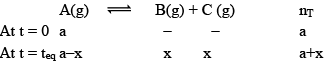You will easily get these expressions in the same way we did in last.

Here, no. of moles increases at equilibrium i.e. from a to (a+x). Hence PT also increases and becomes constant at equilibrium as shown below.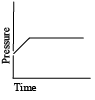Case III: Homogeneous and Δng < 0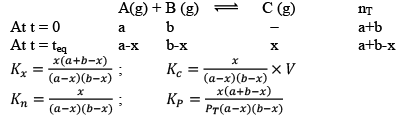This graph clearly resembles that first pressure is decreasing and then becomes constant at equilibrium.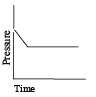1. Synthesis of Hydrogen Iodide:

Suppose ‘a’ moles of H2 and ‘b’ moles o f I2 are heated at 444°C in a closed container of volume ‘V’ litre and at equilibrium, 2x moles of HI are formed.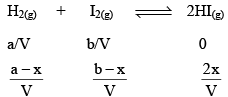Initial concentration (mol L–1)

Equilibrium concentration (mol L–1)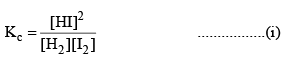Substituting the equilibrium concentrations of H2, I2 and HI in equation (i), we get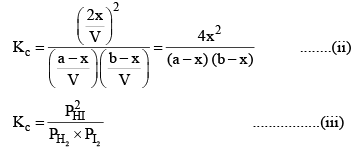Suppose the total pressure of the equilibrium mixture at 444°C is P, then

PHI = mole fraction of HI × Total pressure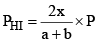Similarly,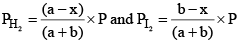Substituting these values in equation (iii) we get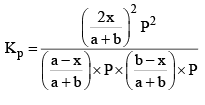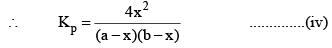So, one can see from equations (iii) and (iv), that

Kp = K

This is so, because Δn = 0 for the synthesis of HI from H2 and I2.

2. Thermal Dissociation of Phosphorus Pentachloride

PCl5(g) dissociates thermally according to the reaction,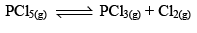Let us consider that 1 mole o f PCl5 has been taken in a container of volume V litre and at equilibrium x moles of PCl5(g) dissociates. Thus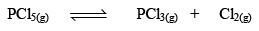Init ial concentration (mo l L–1) 1                        0              0                 0

Equilibrium concentration (mol L–1)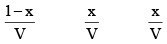According to law of mass action, at constant temperature,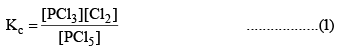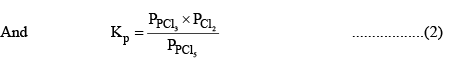Substituting the values of equilibrium concentration, in equation (1), we have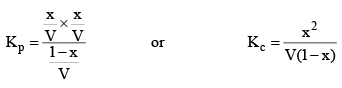Now, total number of moles at equilibrium = 1 – x + x + x = 1 + x

Mole fraction of PCl3 = Mole fraction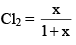And mole fraction of PCl5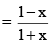Suppose total pressure at equilibrium is P, then we have from equation (2),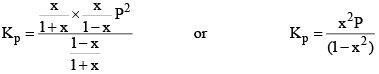Similarly, we can apply law of mass action on any reaction at equilibrium.

6. Equilibrium Constant for Heterogeneous Equilibria

The equilibrium which involves reactants and products in different physical states. The law of mass action can also be applied on heterogenous equilibria as it was applied for homogenous equilibria (involving reactants and products in same physical states).

(i) Thermal Dissociation of Solid Ammonium Chloride:

The thermal dissociat ion of NH4Cl(s) takes place in a closed container according to the equation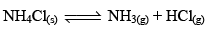Let us consider 1 mole of NH4Cl(s) is kept in a closed container of volume ‘V’ litre at temperature TK and if x mole of NH4Cl dissociates at equilibrium then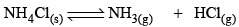Initial moles                           1               0            0

Moles at equilibrium             1- x          x             x

Applying law of mass action, Kc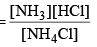As NH4Cl is a pure solid, so there is no appreciable change in its concentration. Thus,

K= [NH3] [HCl]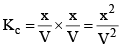And Kp =PNH* PHCl

(ii) Thermal Dissociation of Ag2CO3:

Ag2CO3(s) dissociates thermally according to the equation.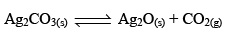Applying law of mass action, at constant temperature, we get,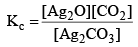Now, let us consider that 1 mole of Ag2CO3(s) is heated in a closed container of volume V and x mo l of Ag2CO3(s) dissociates at equilibrium, then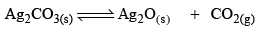Initial moles                                    1             0          0

Equilibrium moles                          1 - x        x           x

Now, as Ag2CO3 and Ag2O are solids, so their concentration can be assumed to be constants Thus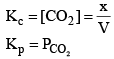7. Significance of magnitude of equilibrium constant:

• A very large value of Kc or Kp signifies that the forward reactions has gone to completion or very nearly so.
•  A very small value o f Kc or Kp signifies that the forward reaction has not occur to any significant extent.
•  If the numerical value of Kc or Kp is neither very large or very small, a reaction is most likely to reach a state of equillibrium in which both reactants and products are present

8. Predictions of direction of the reaction: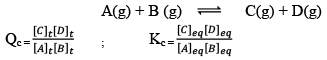QC is called React ion Quotient:

QC is the ratio of concentration of products and reactants each raised to their schoitiometric  coefficient as in balanced chemical equation.

Hence, three cases arises: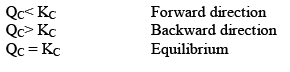Note: While dealing wit h KP and α, take initial moles of reactant as 1 because ultimately they will be cancelled out.

9. Extent of Reaction:

Magnitude of equilibrium constant tells us the extent of reaction. It resembles the extent by which the reactant has been reacted or product has been formed at equilibrium.

Case I: If equilibrium constant (say KC) is very-very small.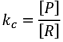(Concentration of product is very low and concentration of reactant is very high) It clearly explains that numerator is very small and denominator is very-very high.

Hence, by this we can infer that reaction has a very low approach in forward direction and reactions has just started.

Case II: If equilibrium constant (i.e. KC) is very-very high.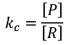(Concentration of product is very high and concentration of reactant is very low).

Hence, it explains that reaction has almost completed.

Illustration: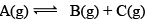Initial moles of reactant is 1 mole and volume of the container is 1L. Calculate the moles of B(g) at Equilibrium Kc = 10–50

Solution: Since the value of KC is very low.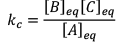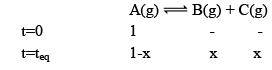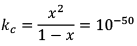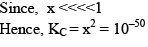x = 10–25

Illustration: If initially 1 mole of all the species present one taken in 10 L closed vessel, find equilibrium concentration of each species.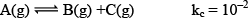Solution: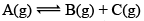At any time concentration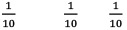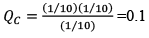Since QC> KC, so reaction will move in backward direct ion to attain equilibrium.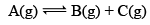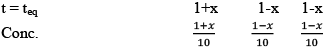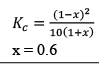Equilibrium concentration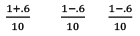Equilibrium concentration of A(g) = 0.16

Equilibrium concentration of B(g) = 0.04

Equilibrium concentration of C(g) = 0.04

Illustration: Calculate KP for the reaction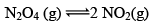When NO2 is 30% dissociated and total pressure at equilibrium is 2 bar.

Solution:

Given, α = 0.3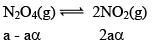We are dealing with KP and α. Hence initial mole of N2O4 = 1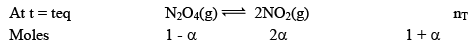Partial Pressure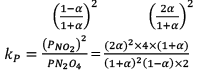Put α = 0.3

KP = 0.79

10. Dealing with degree of dissociation (α)

In terms of moles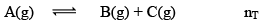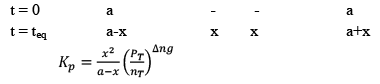In terms of α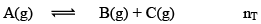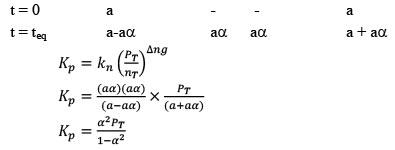Offer running on EduRev: Apply code STAYHOME200 to get INR 200 off on our premium plan EduRev Infinity!

## Physical Chemistry

66 videos|94 docs|32 tests

,

,

,

,

,

,

,

,

,

,

,

,

,

,

,

,

,

,

,

,

,

;